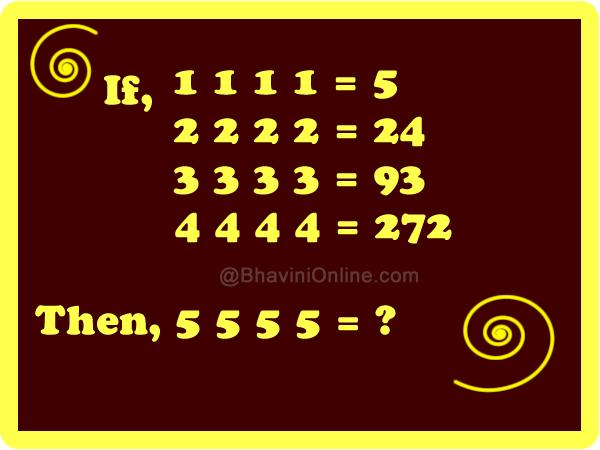# Number Series Riddle: If 1 1 1 1= 5 and 2 2 2 2= 24 Then 5 5 5 5= ?

Check this sequence riddle carefully and find the value of ?

If;

1 1 1 1 = 5

2 2 2 2 = 24

3 3 3 3 = 93

4 4 4 4 = 272

Then;

5 5 5 5= ?So were you able to solve the riddle? Leave your answers in the comment section below.

## 3 Replies to “Number Series Riddle: If 1 1 1 1= 5 and 2 2 2 2= 24 Then 5 5 5 5= ?”

1. Emily Zhang says:

you can just do
1*1*1*1+4=5
2*2*2*2+4*2= 24
3*3*3*3+4*3=93
4*4*4*4+4*4=272
5*5*5*5+4*5=645

2. KB says:

There’s no indication that you can use the numbers twice. This is a shitty riddle.

3. skv says:

645